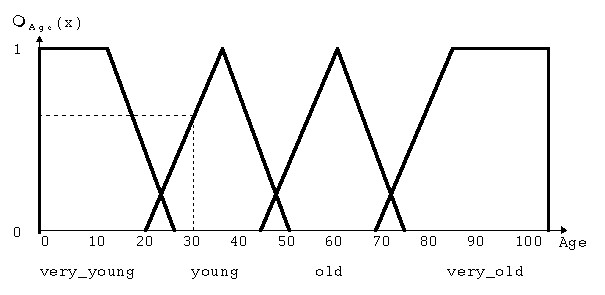# FLIP++

Every problem domain needs suitable datatypes and operators to be done efficiently. StarFLIP++ has the special feature of providing fuzzy datatypes and operators to effectively deal with real-world vagueness. Those are defined in FLIP++ together with all instruments needed for doing defuzzification and inference.

### Operators

FLIP++ defines a wide range of various operators that are needed to implement fuzziness. They include:

• fAND (predefined as: truth(x and y) = minimum (truth(x), truth(y));
• fOR (predefined as: truth(x or y) = maximum (truth(x), truth(y));
• fNOT (predefined as: truth(NOT X) = 1.0 - truth(x);

With the above operators it is possible to model everything that is usually done in boolean logic in the same fashion but within the extended fuzzy-logic domain. As not everyone agrees on how to define fuzzy operators it is possible to adjust them to ones own conception.

Together with the predefined operators it is possible to create new operators that better fit the problem at hand.

### Membership functions

In classical set theory a subset A of a set S maps all elements x of S to the set {0, 1} where 0 means x is not a member of A while 1 means that x is a member of A.

A fuzzy subset F of a set S maps all elements x of S to the interval [0..1] with the meaning of 0 and 1 unchanged but values in-between representing intermediate degrees of membership. This kind of mapping is usually described by a function called the membership function (or fuzzy subset) of F.

As membership functions and logic are generalizations of classical set theory there is no conflict between fuzzy and crisp methods and it is possible to use them both in the system.

One major advantage of fuzzy logic is that it is usually less complicated to define membership functions compared with crisp functions and that they are more intuitively and easier to grasp (just think of the famous example of the definition of tallness of a person ...).

In StarFLIP++ you have to define a membership function for every coinage of a linguistic variable. This can be done in different ways:

1. You can define membership functions as shapes with the x-axis defining the measure (for example temperature) and the y-axis defining the degree of membership in the interval [0..1]. Commonly used shapes like triangular or trapezoidal are already present but again it is possible to define a new, individual shape. The following pictures show first an example of a single membership function and then the membership functions for every coinage of a linguistic variable. As you can see membership functions can be of different shapes and usually overlap. It is also possible to mix fuzzy and crisp functions when appropriate.Fig. 1 shows a typical membership function for the famous age example. The dashed line indicates that Age=30 belongs to the linguistic variable young to a degree of 0.7.

2. The other way to define all membership functions for a linguistic variable is to define a 6-tuple consisting of support range (upper end and lower end of the measure) a median its distribution (around the median) its dilatation (this states the degree of fuzziness in the interval [0..1] with higher fuzziness nearer to 1) and its importance (this parameter lies in the [0..1] interval with higher importances nearer to 1).

### Example

(-100, 100, 0, 0.5, 1, 0.9) defines all membership functions of a linguistic variable with measures in the range of -100 to 100 a median of 0, a distribution of 0.5 around the median, fuzziness 1 and an importance of 0.9 ( the importance belongs of course to the linguistic variable).

Furthermore it is necessary to define the number of coinages for the linguistic variables and their names.

### Rules

To actually be able to get something out of the system we need to specify rules. Rules consist of an antecedent (or premise) and a conclusion and look like this:

### Example

if al_temp is hot and ni_temp is medium then al-ni_heat is acceptable.

al_temp and ni_temp are input variables with hot and medium as membership functions defined on al_temp and ni_temp, respectively. Acceptable is a membership function defined on the output variable al-ni_heat. In the antecedent we determine to what degree the rule applies while in the conclusion the output variable is assigned a value. It is also possible that the conclusion consists of more than one output variable or that there is more than one conclusions for a rule.

In FLIP++ it is also possible to assign a value in the range of [0..1] to a rule to state how accurate (and therefor trustworthy) the rule is

if al_temp is hot and ni_temp is medium then al-ni_heat is acceptable with accuracy 0.7.

### Inferencing in FLIP++

FLIP++ generates the following steps during the inference process:

1. Fuzzification where the degree of truth for the premise of each rule is determined for an actual value.

2. Inference using either max-min or sum-product inferencing. The first method clips off the membership function of the output variable(s) at the computed degree of truth for the premise of the rule (min) and then combines an output fuzzy subset by taking the pointwise maximum for all the fuzzy subsets assigned to a variable (max). The second method scales the membership function of the output variable(s) with the computed degree if truth for the premise of the rule (product) and then combines an output fuzzy subset by taking the pointwise sum for all the fuzzy subsets assigned to a variable (sum).

3. Defuzzification converts a fuzzy output set to a crisp number. From the various methods in use FLIP++ uses the CENTROID method at this time, though it is planned to incorporate other popular methods as well as allowing the user to introduce own methods.

(c)1996 Andreas Raggl, Mazen Younes, Markus Bonner, Wolfgang Slany